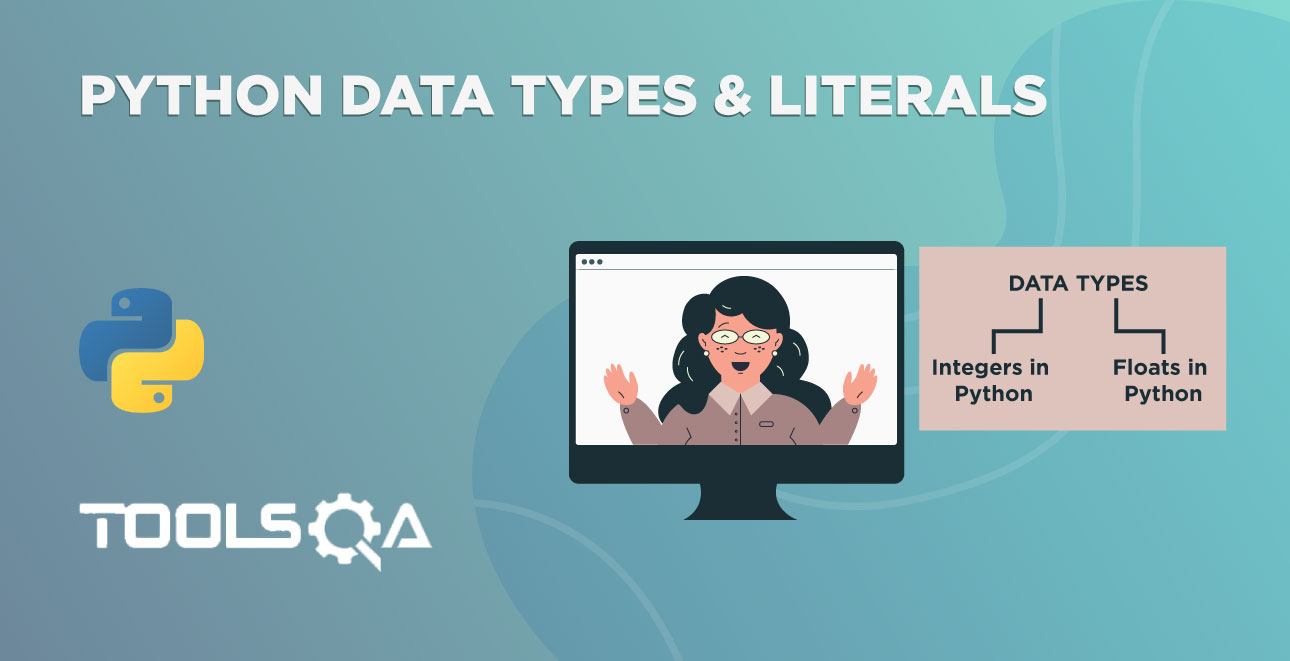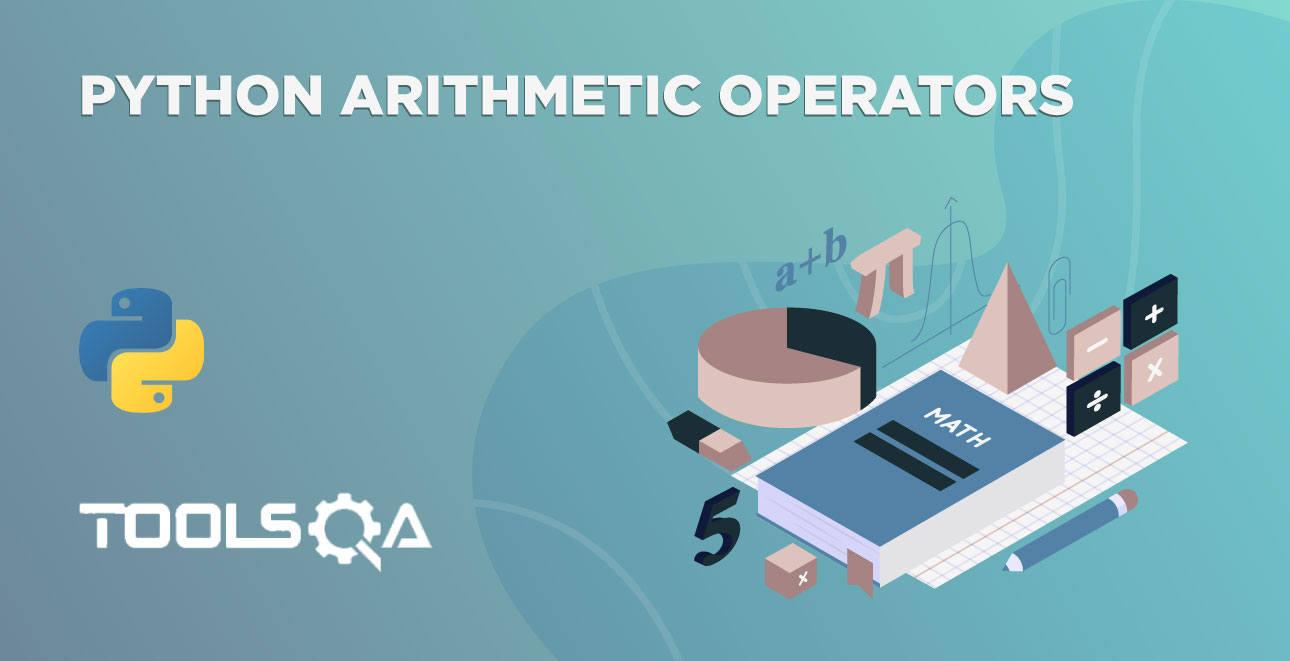We have seen the int and float data types in the previous tutorial. However, there are more Python Literal and Data Types. Consequently, in this tutorial, we are going to see the remaining data types of Python. Let's see the table of contents of the tutorial.

• Python Literal - Character
• chr() and ord() functions
• Python Literal - String
• str() function
• Python Literal - Boolean
• bool() function

## Python Literal - Characters

To start with, Char (or characters) is not a data type in Python (though it is in other languages like Java). From Python's perspective, it is merely a String which is of one character. However, there are some cool character functions and concepts. Therefore, let's discuss it separately from the string.

Computers only understand the numbers (binary). What if we want to represent characters like A, @, \$? We want numbers to represent each character. Additionally, we have an ASCII table that maps each character to a number. ASCII is a character encoding standard for digital devices. Its full form is American Standard Code for Information Interchange. Additionally, each character in the table has a unique value. For example, 65 represents A, and 97 represents a.

Similarly, Python follows the same ASCII table for the representation of characters. And we don't have any data type as a character in Python. It will consider any character or set of characters that are surrounded by quotes as strings. Additionally, different numbers to represent Upper case letters in the ASCII table are:

Number Character
65 A
66 B
67 C
68 D
69 E
70 F
71 G
72 H
73 I
74 J
75 K
76 L
77 M
78 N
79 O
80 P
81 Q
82 R
83 S
84 T
85 U
86 V
87 W
88 X
89 Y
90 Z

We have a method chr() in Python to convert a number into the respective character. Additionally, ord() method work opposite to chr(). Let's see how to work with these functions.

Moreover, you can see the list of characters and corresponding values on the website.

### chr() and ord() functions

We have already discussed the characters in the tutorial. What if you want to know the ASCII value of a character? What will you do if you want to find out the corresponding character of an ASCII value? See the answers to questions.

We can use the chr() function to convert an ASCII value into a character. We have seen some sample characters and their values in the above table. Therefore, let's talk about the chr() function.

chr() function takes only one argument and returns the corresponding character. Moreover, the number that we will pass must be in the range from 0 to 1114111. Maybe most of the characters won't be visible in the PyCharm. Additionally, you can find the visible and most used characters on the site. Let's see how to get the character using its number with the chr() function.

``````# converting the 65 to character
print(chr(65))
``````

The number that we have given is the ASCII value of A. Therefore; the output is A.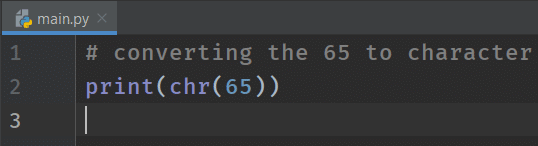Similar to the chr(), we have ord() function that works opposite to the chr(). In other words, the ord() function takes any character and returns the ASCII value of the given character. For example, if you give character A to the ord(), then it returns the number 65 - ASCII value of A.

``````# getting the ASCII value of A
print(ord('A'))
``````

As a result, the output of the above code is 65.## Python Literal - Strings

Text that has single or double quotes at the starting and ending is known as a string. Examples of strings are "ToolsQA," '759', etc. Moreover, there is no particular representation for strings in Python. We can use the examples directly in Python programs. Let's practice some coding.

Write the following code in the IDE and run it.

``````# string
print("ToolsQA")
``````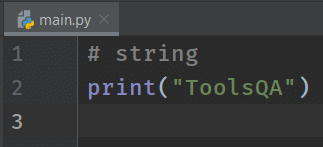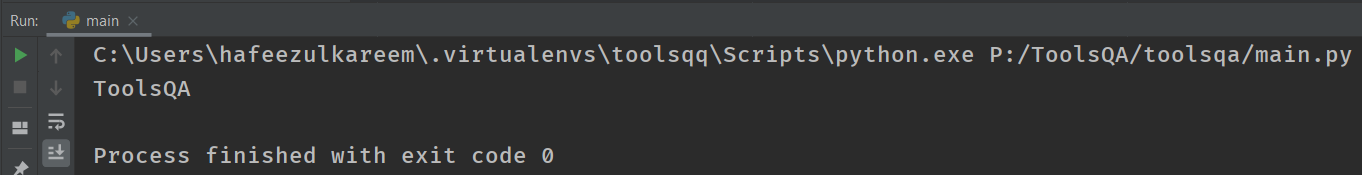Consequently, the output of the above code is ToolsQA. Let's see the same example with single-quotes.

``````# string
print('ToolsQA')
``````### Can we print strings without any content?

Of course, Yes! They are called empty strings. We can use them in coding as well. Moreover, they neither represent anything nor print anything. In addition to this, you will find the usage of empty strings when you have good knowledge of programming.

For now, let's see what happens when we print empty strings?

``````# empty strings
print("")
print('')
``````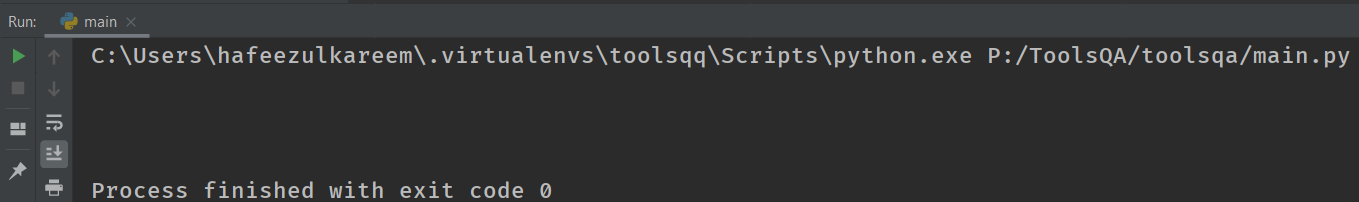We got nothing in the output console. That's what we expected.

### What if we want to print a string with double or single?

For example, I want to print the text "ToolsQA" is one of the great websites to learn programming. We have two ways to achieve the result. First, as we have already seen a method using the escape character, let's see the second way to accomplish this.

Another method is to use other quotes that haven't applied to enclose the string. In other words, whenever you want quotes in the output string, then use the quotes inside the string that haven't used to enclose the same string. Let's see the example to get clarity.

``````# string with quotes
print('"ToolsQA" is one of the great websites to learn programming.')
``````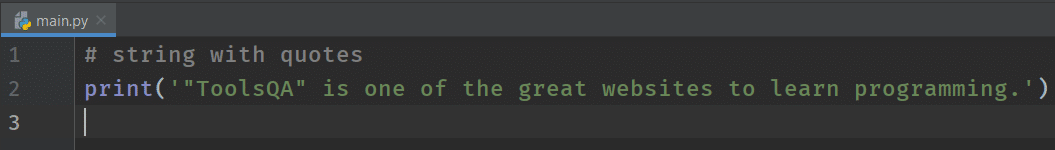Additionally, if you want single quotes in the output, then swap the quotes in the above code as follows.

``````# string with quotes
print("'ToolsQA' is one of the great websites to learn programming.")
``````

Play with the strings using single and double quotes, as mentioned in the examples. Subsequently, we will learn a lot about the strings in the upcoming tutorials. It is to get you started with the concepts.

### str()

str() is similar to the functions int and float. Additionally, it helps to convert objects into a string. And it takes one or zero arguments. In other words, if we don't pass anything to the str() function, then it returns an empty string. Let's see what happens if we don't pass anything to the str function.

``````# str without any arguments
print(str())
``````

As a result, the function str without any arguments returns an empty string.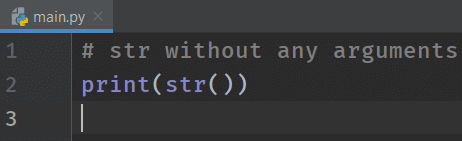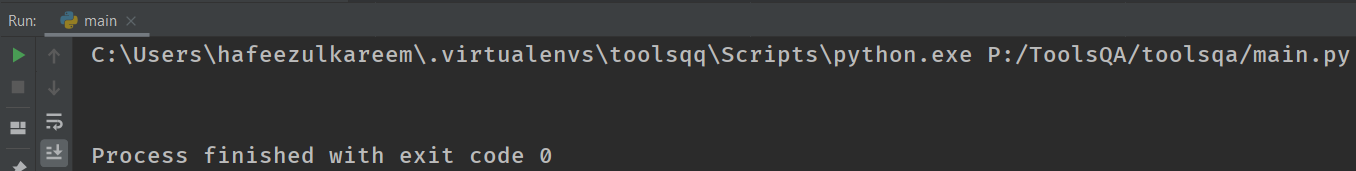See an example of converting the integer to a string. We can directly pass the integer to the str function.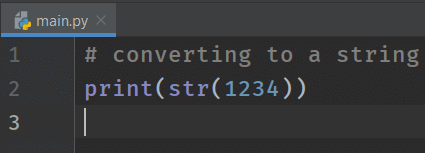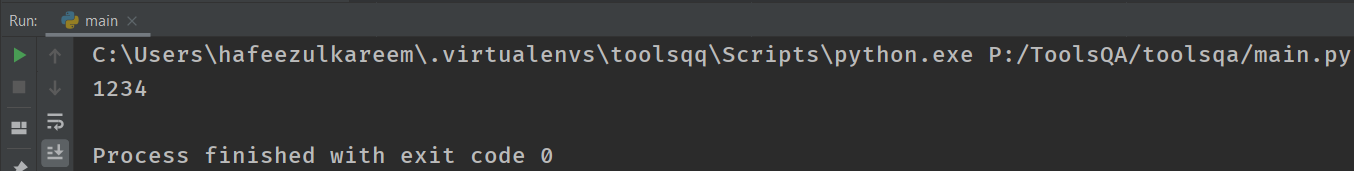We got the output as 1234. But how do you confirm that it is a string? Let's see some more examples to make sure that the result of the str function is a string.

Execute the following program in your IDE.

``````print(1234 + 1)
``````

Consequently, we will get the number 1235 as output.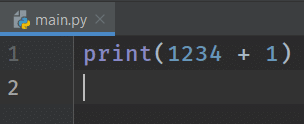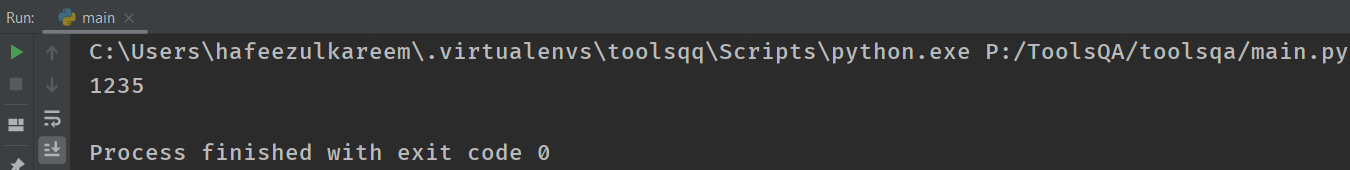Now, run the following program.

``````print(str(1234) + 1)
``````

Did you get the output? No! But why? First, let's see what's the error is and then the reason behind it.The error that Python threw on us is can only concatenate str (not "int") to str. In other words, it means that we can't add an integer to a string. Just remember that we can't add a string to an integer in Python. Therefore, that is the reason why it shows us an error. Conclusively, we can say that the result of the str function is a string.

How to get rid of that error? We can solve it by knowing the meaning of concatenate in Python.

Search and see the general meaning of concatenate. In simple words, concatenate means link together. That is to say; Python links one string at the end of another string using the operator +. Let's see how to rectify the above error using it.

Firstly, modify the code of the previous program as follows.

``````print(str(1234) + "1")
``````

After that, guess the output. And check it with the actual output.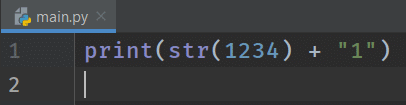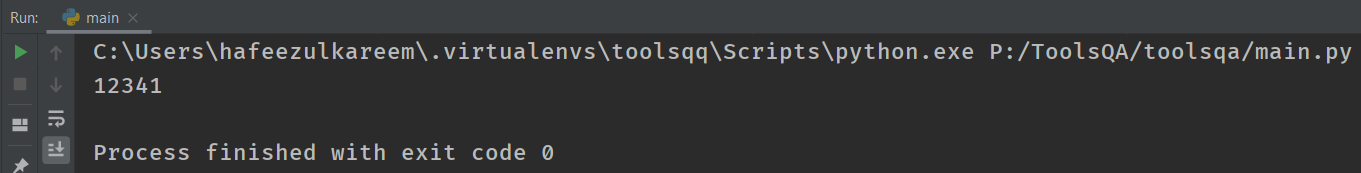Code_11Finally, we didn't get any errors. Moreover, Python links the second string to the end of the first string. We will talk about it in detail in the later tutorials.

## Python Literals - Boolean

Python literal Boolean has two values. One is True, and another one is False. In any programming language, Booleans have only two values. In the same vein, Python language also exhibits the same properties. Any non-zero value is True, and the value zero is False. Additionally, 1 and 0 represent True and False, respectively.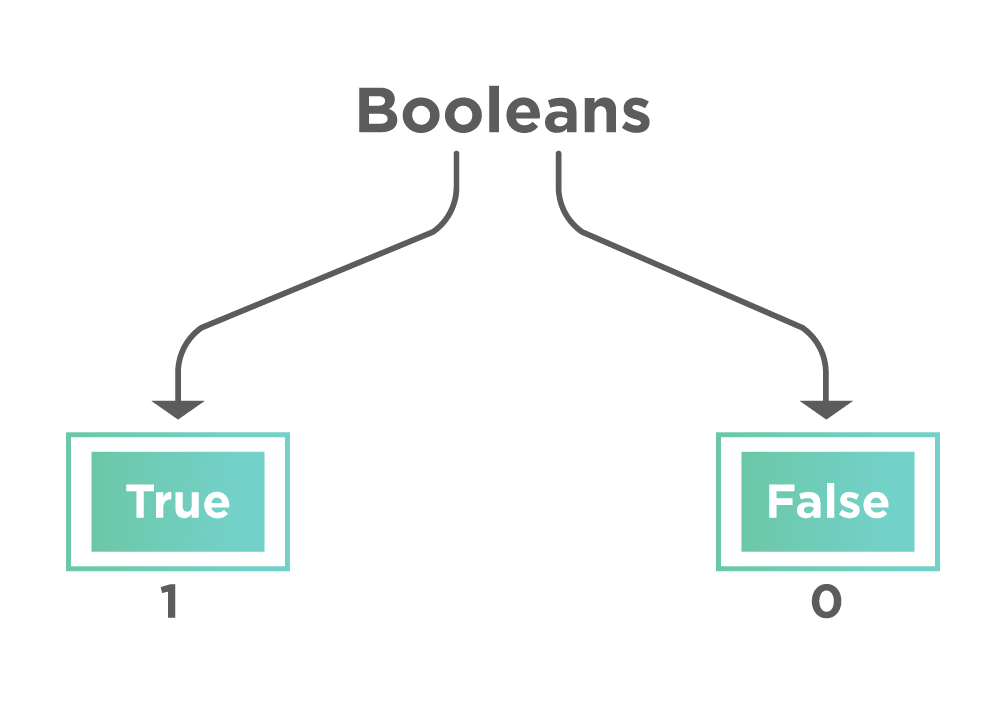In addition to the above, Python has a method called bool() to convert any object into a boolean value. Let's examine the process in detail.

### bool()

The function bool() returns either True or False based on the argument. Moreover, it takes one or zero arguments. That is to say, if we didn't pass anything to the bool(), then it will return False. Moreover, for any non-zero value bool() returns True. Let's see some examples.

``````# boolean value of a non-zero
print(bool(5))
``````

The output of the above code is True. We have passed a non-zero value. Therefore, it returns True.Guess what will be the output of the following code.

``````# boolean value of zero
print(bool(0))
``````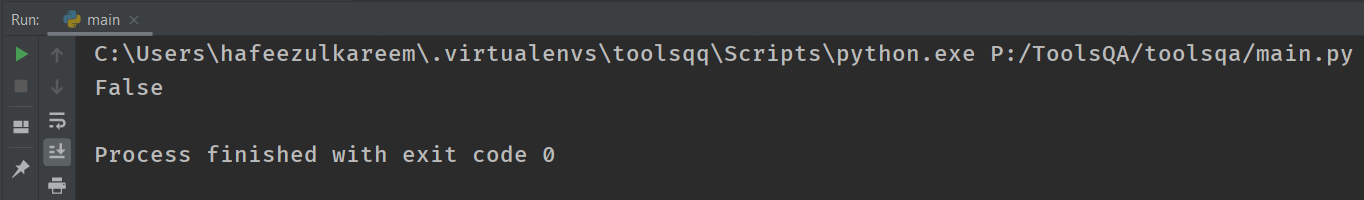Some quick examples where the function bool() returns True.

``````# float
print(bool(7.59))

# strings
print(bool("ToolsQA"))
print(bool('ToolsQA'))
``````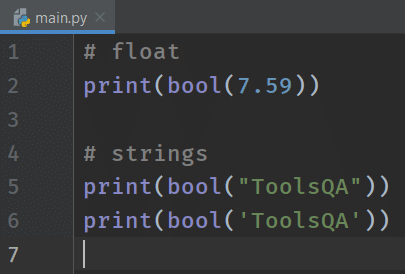We have different situations where the function bool() returns False. Let's see some quick examples.

``````# zero as float
print(bool(0.0))

# empty strings
print(bool(""))
print(bool(''))
``````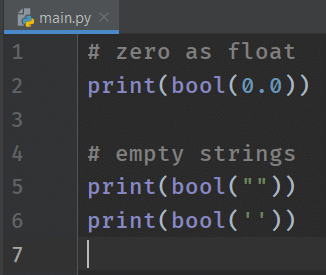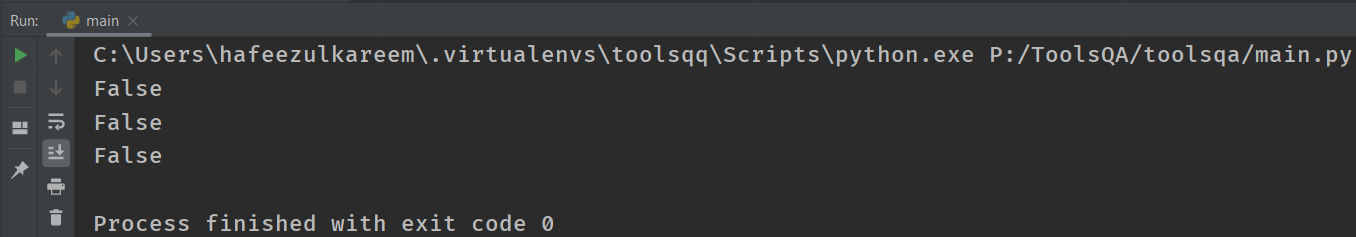We have a lot of other cases where the bool() returns False. We will see them in future tutorials.

Previously I have stated that True is 1, and False is 0. How can we prove it? We can prove it by converting the True and False to integers using the int() function. Let's see the code for the same.

``````# converting True and False to integers
print(int(True))
print(int(False))
``````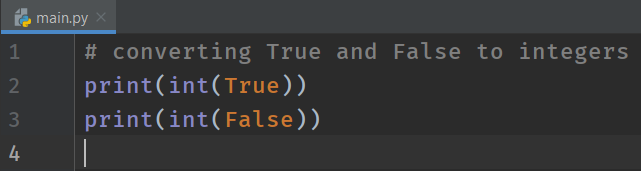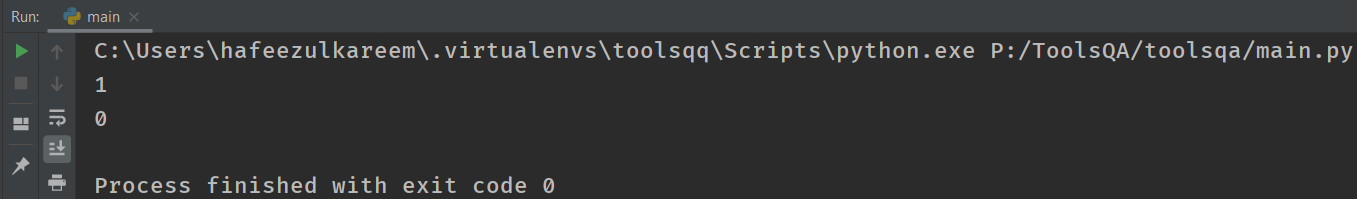We got the output as we expected. 1 for True and 0 for False.

When do we get the output as a boolean value in Python? There's a lot of stuff around the answer. We will learn them slowly. For a glimpse, if you compare one number with another, you will get a boolean value as a result in Python. Let's take the example 3 > 2. Is it True or False? Absolutely, True. Similarly, we can do it for the less than symbol as well.

We know that True is 1, and False is 0. Guess the output of the following statements.

``````print(True < False)
print(True > False)
``````

That's it for the tutorial.

## Python Literal - Key Takeaways

• Programming languages use ASCII value to represent characters.
• Moreover, chr(ascii_value) gives you the corresponding character of the ascii_value. Similarly, ord(character) gives you the corresponding ASCII value of the character.
• Additionally, the set of characters enclosed in single or double quotes is known as a string. Example: "ToolsQA".
• The corresponding function for strings in Python is str(). It converts an object into a string.
• Moreover, we have a boolean data type, which has two values True and False. True = 1 and False = 0 .
• bool() functions convert any object into a boolean.
• Additionaly, printing bool() Function would result in True or False based on Argument. print(bool(2>1)) will result in True while print(bool(2<1)) will result in False.
• Also, printing empty bool() function will always result in False.

## Python Literal - Practice Yourself

#Problem 1

What is the output of the code: chr(48)

What is the output of the code: ord('1')

#Problem 2

What is the output of the expression: str(45) + 45

# Problem 3

What is the output of the expression: str(45) + "45"

# Problem 4 b

What is the output of the code: bool(int())

What's the output of the code: bool(float())

What is the output of the code: bool(str())

# Problem 5

What is the output of the code: bool(int(1))

What's the output of the code: bool(float(1))

What is the output of the code: bool(str("ToolsQA"))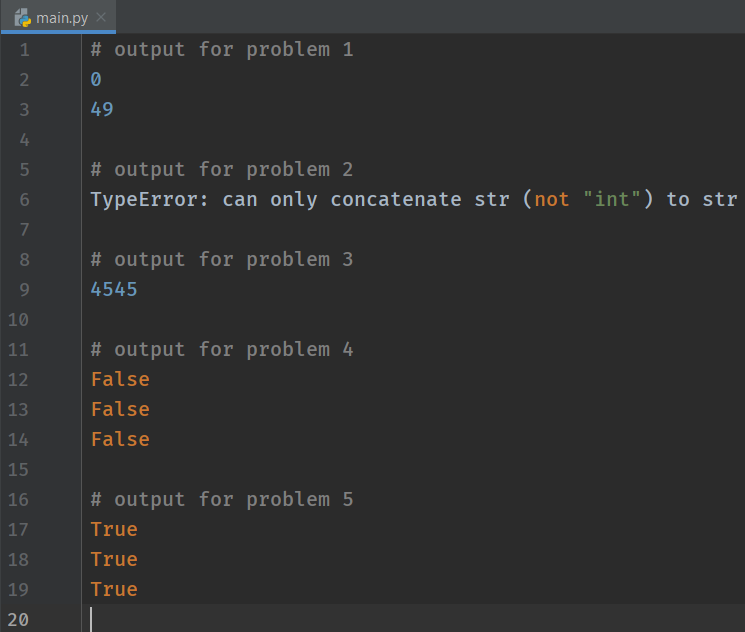## What Next?

Hurray! To conclude, we have completed all the data types in Python & Python Literal. We know the mathematical operations like addition, subtraction, multiplication, etc. In other words, they are called Arithmetic operations. How can we perform those operations in Python?

Consequently, we will have a set of arithmetic operators to perform the same operations in programming languages. Therefore, we are going to look at the arithmetic operations in the next tutorial.

As always, keep practicing all the examples that we have seen in this tutorial

Happy Coding :)